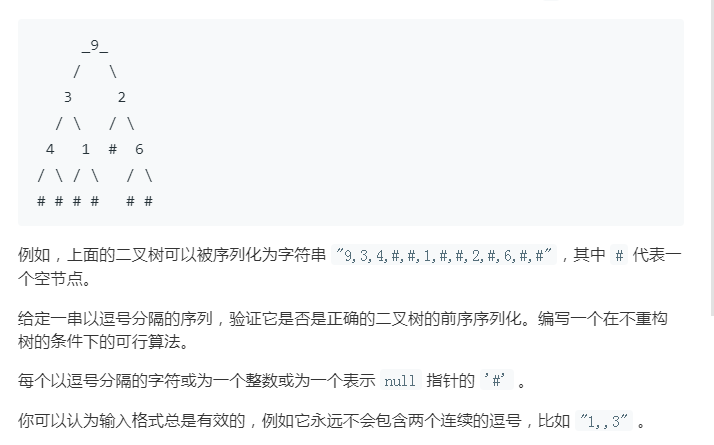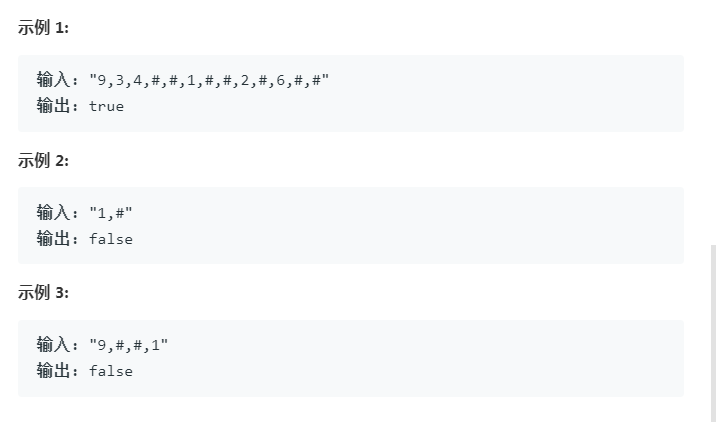# LeetCode_331验证二叉树的前序序列化class Solution {
/*
* 基本思路：
* 1、这种方法表示的二叉树，每个节点的左右孩子节点必存在（因为不存在用#表示了，相当于存在）
* 2、将字符串拆分，从后向前压栈
* 3、遇到#直接压栈
* 4、遇到数字x，弹出两个栈顶元素，并将这个数字x压栈
* 5、如果是合法的，最后栈中只会剩一个根元素
*/
public boolean isValidSerialization(String preorder) {
String[] ss = preorder.split(",");
int len = ss.length;
if(len==1){
if(ss.equals("#"))
return true;
return false;
}
Stack<String> stack = new Stack<String>();
for(int i=len-1;i>=0;i--){
String s = ss[i];
if(stack.empty()){
stack.push(s);
continue;
}
if(s.equals("#")){
stack.push(s);
}else{
if(stack.size()<2) return false;
String s1 = stack.pop();
String s2 = stack.pop();
stack.push(s);
}
}
if(stack.size()==1)
return true;
return false;
}
}


©️2019 CSDN 皮肤主题: 大白 设计师: CSDN官方博客